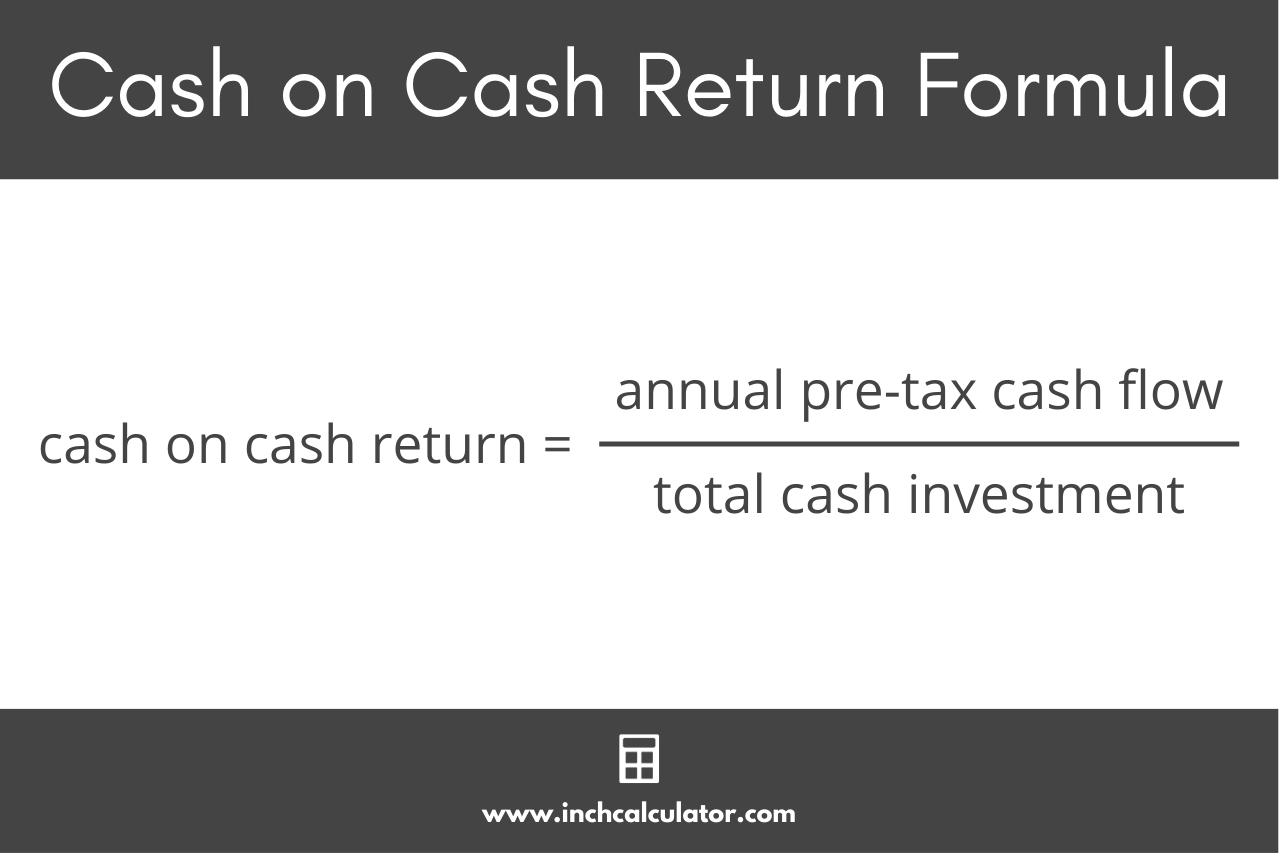# Cash on Cash Return Calculator

Calculate the cash on cash return for an investment using the calculator below.

\$
Income
\$
\$
Expenses
\$
\$

## Cash on Cash Return:

Learn how we calculated this below

## How to Calculate Cash on Cash Return

Cash on cash return is a metric used to evaluate the rate of return and profitability of a real estate investment. Cash on cash return is a ratio of the property’s cash flows to the total cash investment, expressed as a percentage.

This metric is useful to help determine whether a property can generate a positive cash flow and is worth investing in.

To calculate the cash on cash return for a property, you need to follow a few simple steps.

### Step One: Calculate the Cash Flow

The first step in calculating cash on cash return is to determine the cash flow. The cash flow is the difference between the income generated by the property and the total expenses, including operating expenses and debt payments.

This is similar to net operating income but is slightly different. The cash flow for calculating cash on cash return includes debt payments, whereas NOI does not.

To calculate cash flow, estimate the total annual rent income and the total annual expenses. Then, subtract the total annual expenses from the total rental income. The result is the cash flow.

cash flow = total income – total expenses

It’s important to note that the cash flow is a pre-tax cash flow and does not account for taxes or property depreciation.

### Step Two: Determine the Total Cash Investment

The next step in calculating cash on cash return is to determine the total investment. This is the total equity, or the amount of up-front cash invested in the property, including the cost of debt financing.

### Step Three: Calculate Cash on Cash Return

Once you know the annual pre-tax cash flow and the total cash investment, you can calculate the cash on cash return using a simple formula.

cash on cash return = annual pre-tax cash flow / total cash investment

Thus, the cash on cash return is equal to the annual pre-tax cash flow divided by the total cash investment, expressed as a percentage.This formula is very similar to the return on equity formula, but a key difference between them is that cash on cash return uses pre-tax cash flow rather than post-tax net income.

return on equity = net income / equity (cash investment)

It’s also important to note that the cash on cash return only takes into account the property’s cash flow and does not include any appreciation in value or account for the sale price of the investment, including associated costs such as commission.

## Cash on Cash Return vs. IRR

Cash on cash return is also very similar to the internal rate of return, however, cash on cash return is a measure of the return over a single period (one year). The metric only considers the initial investment and first year’s cash flow and does not apply the concept of the time value of money.

The internal rate of return, on the other hand, considers the cash flows over multiple periods and accounts for the time value of money by discounting cash flows.

### Is cash on cash return the same as ROI?

No, cash-on-cash return does not measure some additional factors that the return on investment formula does, such as appreciation or depreciation in property value, taxes, and the total return on the investment, not just the cash.

Return on investment is a measure of the total rate of return on an investment property, including debt and cash, but cash on cash is only a measure of the return on the actual cash invested.

### Is cash on cash return a percentage?

Yes, cash on cash return is a ratio of a property’s pre-tax cash flow to the total cash investment, and is expressed as a percentage.

### Can cash on cash yield be negative?

Yes, a negative cash on cash return can happen as a result of having more expenses than income for the property. Low rent or vacancy can contribute to a negative cash flow, which leads to a negative cash on cash yield.

However, this does not mean that the return on investment will also be negative. Recall that cash on cash return does not account for appreciation in value, debt leveraging, or multiple years’ cash flows, which make up the total return on investment.

### How is cash on cash return different from the cap rate?

The cap rate is a ratio of the net operating income to the property’s value. Cash on cash return, on the other hand, is a ratio of the cash flow (including debt payments) to the cash investment.

Cash on cash measures the income in relation to the cash investment, while the cap rate measures income in relation to the value of the property.